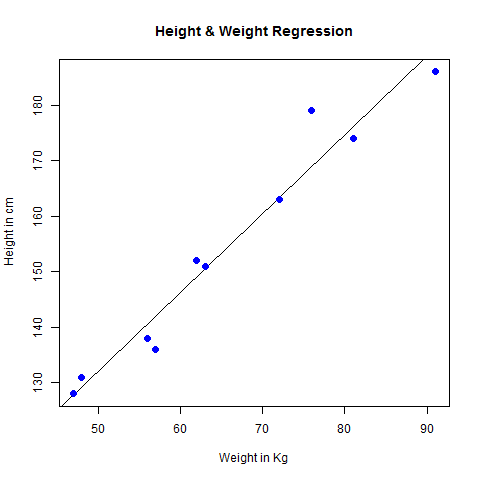> R语言中文手册 > R语言 线性回归

```y = ax + b
```

• y是响应变量。

• x是预测变量。

• ab被称为系数常数。

## 建立回归的步骤

• 进行收集高度和相应重量的观测值的样本的实验。

• 使用R语言中的lm()函数创建关系模型。

• 从创建的模型中找到系数，并使用这些创建数学方程

• 获得关系模型的摘要以了解预测中的平均误差。 也称为残差。

• 为了预测新人的体重，使用R中的predict()函数。

### 输入数据

```# Values of height
151, 174, 138, 186, 128, 136, 179, 163, 152, 131

# Values of weight.
63, 81, 56, 91, 47, 57, 76, 72, 62, 48
```

## LM()函数

### 语法

```lm(formula,data)
```

• 公式是表示xy之间的关系的符号。

• 数据是应用公式的向量。

### 创建关系模型并获取系数

```x <- c(151, 174, 138, 186, 128, 136, 179, 163, 152, 131)
y <- c(63, 81, 56, 91, 47, 57, 76, 72, 62, 48)

# Apply the lm() function.
relation <- lm(y~x)

print(relation)
```

```Call:
lm(formula = y ~ x)

Coefficients:
(Intercept)            x
-38.4551          0.6746
```

### 获取相关的摘要

```x <- c(151, 174, 138, 186, 128, 136, 179, 163, 152, 131)
y <- c(63, 81, 56, 91, 47, 57, 76, 72, 62, 48)

# Apply the lm() function.
relation <- lm(y~x)

print(summary(relation))
```

```Call:
lm(formula = y ~ x)

Residuals:
Min      1Q     Median      3Q     Max
-6.3002    -1.6629  0.0412    1.8944  3.9775

Coefficients:
Estimate Std. Error t value Pr(>|t|)
(Intercept) -38.45509    8.04901  -4.778  0.00139 **
x             0.67461    0.05191  12.997 1.16e-06 ***
---
Signif. codes:  0 ‘***’ 0.001 ‘**’ 0.01 ‘*’ 0.05 ‘.’ 0.1 ‘ ’ 1

Residual standard error: 3.253 on 8 degrees of freeDOM
Multiple R-squared:  0.9548,    Adjusted R-squared:  0.9491
F-statistic: 168.9 on 1 and 8 DF,  p-value: 1.164e-06
```

## predict()函数

### 语法

```predict(object, newdata)
```

• object是已使用lm()函数创建的公式。

• newdata是包含预测变量的新值的向量。

### 预测新人的体重

```# The predictor vector.
x <- c(151, 174, 138, 186, 128, 136, 179, 163, 152, 131)

# The resposne vector.
y <- c(63, 81, 56, 91, 47, 57, 76, 72, 62, 48)

# Apply the lm() function.
relation <- lm(y~x)

# Find weight of a person with height 170.
a <- data.frame(x = 170)
result <-  predict(relation,a)
print(result)
```

```       1
76.22869
```

### 以图形方式可视化回归

```# Create the predictor and response variable.
x <- c(151, 174, 138, 186, 128, 136, 179, 163, 152, 131)
y <- c(63, 81, 56, 91, 47, 57, 76, 72, 62, 48)
relation <- lm(y~x)

# Give the chart file a name.
png(file = "linearregression.png")

# Plot the chart.
plot(y,x,col = "blue",main = "Height & Weight Regression",
abline(lm(x~y)),cex = 1.3,pch = 16,xlab = "Weight in Kg",ylab = "Height in cm")

# Save the file.
dev.off()
```